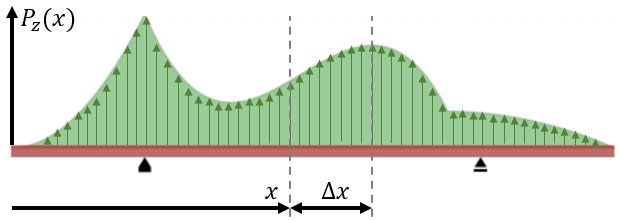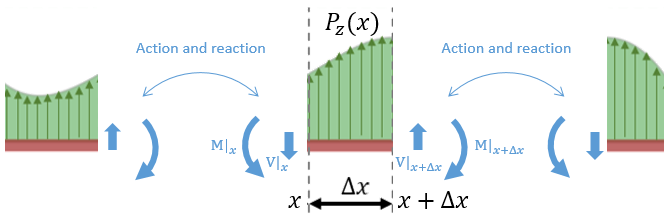# Relationship between shear force and bending moment¶

The analysis in the section above was restricted to point loads in order to keep it simple. However, it applies universally for distributed loads as well, as we are going to see next.

It may not be obvious at first sight, but the functions corresponding to shear force $$\mathbf{V(x)}$$ and bending moment $$\mathbf{M(x)}$$ are intimately correlated (i.e. you can use one of them for calculating the other one). We are going to prove this by performing the same analysis explained above to a differential beam segment of length $$\Delta x$$.

By this point, our analysis methodology should be clear. It consists of the following steps:

1. Choosing an arbitrary sector of a beam.
2. Drawing a free-body diagram of the partition.
3. Applying Newton’s first law to the free-body diagram.

Let’s consider an arbitrarily loaded beam as shown in the figure below, where $$\mathbf{P_z}(x)$$ is an arbitrarily distributed load applied to the beam.Generic problem consisting of a beam under an arbitrarily distributed load $$\mathbf{P_z}(x)$$

Let’s zoom-in and draw a free-body diagram of a given beam segment $$[x_0 \leq x \leq x_0+\Delta x]$$.Free body diagram of a differential segment of the beam

The equilibrium of vertical forces yields then the following:

$\begin{split}\begin{array}{rrl} & \mathbf{\sum{F_z}} &= 0 \\ & V(x + \Delta x) -V(x) + \overline{\mathbf{P_z}}(x) \Delta x &= 0 \\ & \cfrac{V(x + \Delta x) -V(x)}{\Delta x} &= -\overline{\mathbf{P_z}}(x) \\ \lim_{\Delta x \to 0} & \boxed{\cfrac{dV(x)}{dx} = -\mathbf{P_z}(x)} \end{array}\end{split}$

which in words means that the rate of change of the shear force is equal to (minus) the value of the distributed load acting on a given x-coordinate.

Note that in the expression above, $$\overline{\mathbf{P_z}}(x)$$ represents the average value of $$\mathbf{P_z}(x)$$ in the given interval. As $$\Delta x$$ goes to zero, $$\overline{\mathbf{P_z}}(x)$$ converges to $$\mathbf{P_z}(x)$$.

The equilibrium of moments can be written as:

$\begin{split}\begin{array}{rrl} & \mathbf{\sum M|_{x + \Delta x}} &= 0 \\ & M(x + \Delta x) -M(x) - V(x) \Delta x + (\overline{\mathbf{P_z}}(x) \Delta x \cdot \frac{\Delta x}{2}) &= 0 \\ & \cfrac{M(x + \Delta x) -M(x)}{\Delta x} + \underbrace{(\overline{\mathbf{P_z}}(x) \Delta x \cdot \frac{\Delta x}{2})}_{\Delta x^2\to 0} &= V(x) \\ \lim_{\Delta x \to 0} & \boxed{\cfrac{dM(x)}{dx} = V(x)} \end{array}\end{split}$

which presents explicitly the relationship between $$M(x)$$ and $$V(x)$$: the rate of change of the bending moment at a given point is equal to the shear force at that point.

Ok, that was it. For a worked example, take a look at the next section: Beam with two point loads and two distributed loads.